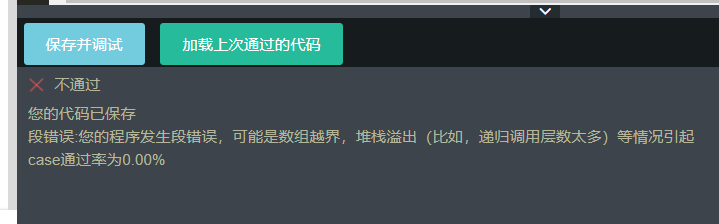# 牛客2018校招真题——拼多多.最大乘积——题解+代码实现

### 一、题目

``无序整数数组A[n]``

``满足条件的最大乘积``

``````4
3 4 1 2``````

``24``

### 二、题解思路

• 方法一题解思路：先建立一个一维vector向量，for循环存要输入的数组A[n]，使用sort对数组进行升序排序。分析可知，最大乘积出现在排序后数组的：
1、最后三个的乘积：n-1、n-2、n-3
2、最前面两个和最后一个的乘积：0、1、n-2
使用long long类型的目的就是三个数乘积后的结果可能会超出int或者long的存储范围，因为试过int，只能通过22%。
• 方法二题解思路：找出数组中的第一大、第二大、第三大的三个数和数组中的第一小和第二小的两个数，最后答案选择（第一大*第二大*第三大，第一小*第二小*第一大）中的最大者。

### 三、代码实现

• 方法一C++代码实现

注意：牛客测试用例有问题，估计是太少了，这个算法使用sort会有O（nlogn）的时间复杂度，不能满足要求，但能AC，说明牛客设置的测试数据不够严谨。

``````#include<bits/stdc++.h>   //万能的C++头文件，包含了基本上用到的所有头文件
using namespace std;

int main()
{
int n;
cin>>n;  //输入数组大小n
vector<long long> nums(n,0); //建立大小为n的vector数组,一定要这样写，否则会发生段错误（数组越界，堆栈溢出（比如，递归调用层数太多）等情况引起）
for(int i = 0;i<n;i++)
{
cin>>nums[i];   //只有上面vector那样写了，这里才可以这样写
}
sort(nums.begin(),nums.end());   //使用算法中的sort函数对数组进行升序排序
long long temp1 = nums*nums*nums[n-1];     //前两个和最后一个相乘
long long temp2 = nums[n-1]*nums[n-2]*nums[n-3];  //最后三个相乘
if(temp1 >= temp2)
cout<<temp1;
else
cout<<temp2;
return 0;
}``````
• 方法二C++代码实现
``````/*方法二：排序找到最大的三个和最小的两个*/
#include<bits/stdc++.h>
using namespace std;

int main()
{
int n;
cin>>n;
vector<long long> nums(n,0);  //存输入的数组
long long max_1 = 1,max_2 = 1,max_3 = 1,min_1 = 1,min_2 = 1;//第一大、二大、三大、第一小、二小
for(int i = 0;i<n;i++)
{
cin>>nums[i];
if(nums[i] > max_1)
{
max_3 = max_2;
max_2 = max_1;
max_1 = nums[i];
}
else if(nums[i] > max_2)  //已经不满足nums[i] > max_1
{
max_3 = max_2;
max_2 = nums[i];
}
else if(nums[i] > max_3)  //已经不满足nums[i]>max_2
{
max_3 = nums[i];
}
else if(nums[i] < min_1)  //已经不满足nums[i] > max_3
{
min_2 = min_1;
min_1 = nums[i];
}
else if(nums[i] < min_2)   //已经不满足nums[i] < min_1
{
min_2 = nums[i];
}
}
long long result1 = max_1*max_2*max_3;  //三个最大值相乘（第一大、二大、三大）
long long result2 = min_1*min_2*max_1;  //两个最小值（第一小、二小）和第一大值相乘
if(result1 > result2)
cout<<result1;
else
cout<<result2;
return 0;
}``````### 四、知识点

1、int、long、long long取值范围

 unsigned int 0~4294967295（10位数，4e9   ，4*10^9） int -2147483648~2147483647（10位数，2e9， 2^31-1） long（同int） -2147483648~2147483647 unsigned long（同unsigned int） 0~4294967295 long long -9223372036854775808～9223372036854775807     (19位数， 9e18  ，2^63-1） unsigned long long unsigned long long：0～18446744073709551615        (20位数，1e19  ， 2^64-1）

2、万能的C++笔试头文件

3、vector的使用——插入新元素和在原有的旧元素基础上进行赋值操作

• 向vector中添加元素：push_back（）
``````vector<int> nums;
for(int i = 0;i < 20; i++)
{
nums.push_back(i);
}``````
• 注意的细节：只能对vector中已经存在的元素进行赋值（=）或者修改操作，不能对vector中不存在的元素进行赋值修改
``````vector<int> vec;
vec = 1;  //错误！``````

上述就是错误的写法

• 在写程序的时候也出现了这样的情况，当我像这样写的时候就会报错：
``````#include<bits/stdc++.h>   //万能的C++头文件，包含了基本上用到的所有头文件
using namespace std;

int main()
{
int n;
cin>>n;  //输入数组大小n
vector<long long> nums;   //错误的写法，这样写，cin>>nums[i]就会出错，因为vector还没有元素，更谈不上赋值操作
for(int i = 0;i<n;i++)
{
cin>>nums[i];
}
sort(nums.begin(),nums.end());   //使用算法中的sort函数对数组进行升序排序
long long temp1 = nums*nums*nums[n-1];     //前两个和最后一个相乘
long long temp2 = nums[n-1]*nums[n-2]*nums[n-3];  //最后三个相乘
if(temp1 >= temp2)
cout<<temp1;
else
cout<<temp2;
return 0;
}``````;